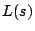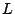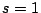# The Riemann Hypothesis and its generalizations

The Riemann Hypothesis foris the assertion that the nontrivial zeros oflie on the critical line. For historical reasons there are names given to the Riemann hypothesis for various sets of-functions. For example, the Generalized Riemann Hypothesis (GRH) is the Riemann Hypothesis for all Dirichlet \$L\$-functions. More examples collected below.

In certain applications there is a fundamental distinction between nontrivial zeros on the real axis and nontrivial zeros with a positive imaginary part. Here we use the adjective modified to indicate a Riemann Hypothesis except for the possibility of nontrivial zeros on the real axis. Thus, the Modified Generalized Riemann Hypothesis (MGRH) is the assertion that all nontrivial zeros of Dirichlet \$L\$-functions lie either on the critical line or on the real axis.

Nontrivial zeros which are very close to the pointare called Landau-Siegel zeros.

Back to the main index for The Riemann Hypothesis.